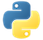# SumPydoc

Sums all the elements within each aggregation.

## Examples

In the following example, we create a pipeline with a `PCollection`. Then, we get the sum of all the element values in different ways.

### Example 1: Sum of the elements in a PCollection

We use `Combine.Globally()` to get sum of all the element values from the entire `PCollection`.

``````import apache_beam as beam

with beam.Pipeline() as pipeline:
total = (
pipeline
| 'Create numbers' >> beam.Create([3, 4, 1, 2])
| 'Sum values' >> beam.CombineGlobally(sum)
| beam.Map(print))``````

Output:

``10``View source code

### Example 2: Sum of the elements for each key

We use `Combine.PerKey()` to get the sum of all the element values for each unique key in a `PCollection` of key-values.

``````import apache_beam as beam

with beam.Pipeline() as pipeline:
totals_per_key = (
pipeline
| 'Create produce' >> beam.Create([
('🥕', 3),
('🥕', 2),
('🍆', 1),
('🍅', 4),
('🍅', 5),
('🍅', 3),
])
| 'Sum values per key' >> beam.CombinePerKey(sum)
| beam.Map(print))``````

Output:

``````('🥕', 5)
('🍆', 1)
('🍅', 12)``````View source codePydoc